StatLect

# Coordinate vector

In a vector space, any vector can be written as a linear combination of a basis. The coefficients of the linear combination are called the coordinates of the vector with respect to the basis.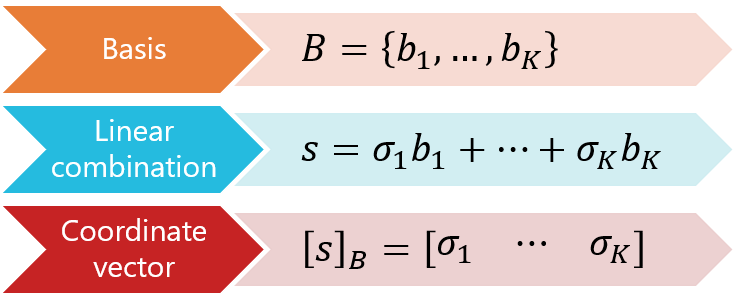## Motivation

Previously, we have provided two definitions of a vector space:

• informal definition: a vector is a finite array of numbers, and a set of such arrays is said to be a vector space if and only if it is closed with respect to taking linear combinations;

• formal definition: a vector space is a set equipped with two operations, called vector addition and scalar multiplication, that satisfy a number of axioms.

The simpler informal definition is perfectly compatible with the more formal definition, as a set of numerical arrays satisfies all the properties of a vector space, provided that vector addition and scalar multiplication are defined in the usual manner and that the set is closed with respect to linear combinations.

We now introduce a new concept, that of a coordinate vector, which makes the two definitions almost equivalent: if we are dealing with an abstract vector space, but its dimension is finite and we are able to identify a basis for the space, then we can write each vector as a linear combination of the basis; as a consequence, we can represent the vector as an array, called a coordinate vector, that contains the coefficients of the linear combination.

Once we have obtained this simple representation, we can apply the usual rules of matrix algebra to the coordinate vectors, even if we are dealing with an abstract vector space.

Not only this is very convenient, but it blurs the differences between the two approaches to defining vectors and vector spaces (at least for the finite-dimensional case).

## Definition

We are now ready to give a definition of a coordinate vector.

Definition Letbe a finite-dimensional linear space. Letbe a basis for. For any, take the unique set ofscalarssuch thatThen, thevectoris called the coordinate vector ofwith respect to the basis.

Note that the uniqueness of the scalarsis guaranteed by the uniqueness of representations in terms of a basis.

## Examples

Let us make some examples.

### Example 1

Letbe a vector space anda basis for it.

Suppose that a vectorcan be written as a linear combination of the basis as follows:Then, the coordinate vector ofwith respect tois### Example 2

Consider the spaceof second-order polynomialswhere the coefficientsand the argumentare scalars.

As we have already discussed in the lecture on linear spaces,is a vector space provided that the addition of polynomials and their multiplication by scalars is performed in the usual manner.

Consider the polynomialsThese three polynomials form a basisforbecause they are linearly independent (no combination of them is equal to zero for any) and they can be linearly combined so as to obtain anyof the form above: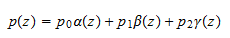The coordinate vector ofwith respect to the basis we have just found isThe addition of two vectors can be carried out by performing the usual operation of vector addition on their respective coordinate vectors.

Proposition Letbe a linear space anda basis for. Let. Then, the coordinate vector ofwith respect to the basis is equal to the sum of the coordinate vectors ofandwith respect to the same basis, that is,Proof

Suppose that the representations in terms of the basis areso that the coordinate vectors are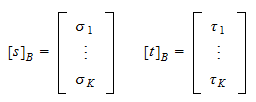By the commutative and distributive properties of vector addition and scalar multiplication in abstract vector spaces, we have that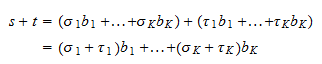Thus, the coordinate vector ofis## Multiplication of coordinate vectors by scalars

The multiplication of a vector by a scalar can be carried out by performing the usual operation of multiplication by a scalar on its coordinate vector.

Proposition Letbe a linear space anda basis for. Letand letbe a scalar. Then, the coordinate vector ofwith respect to the basis is equal to the product ofand the coordinate vector of, that is,Proof

Suppose the representation in terms of the basis isso that the coordinate vector isBy the associative and distributive properties of scalar multiplication in abstract vector spaces, we have thatThus, the coordinate vector ofis## Numeric arrays are coordinate vectors with respect to the canonical basis

When the elements of a linear spaceare one-dimensional arrays of numbers (vectors, in the simplest sense of the term), then they coincide with their coordinate vectors with respect to the standard basis.

Example Letbe the space of allcolumn vectors. Letbe its canonical basis, whereis a vector whose entries are all, except the-th, which is equal to:Take anyThen,is the same as its coordinate vector with respect to the basis, that is,because## Solved exercises

Below you can find some exercises with explained solutions.

### Exercise 1

Letbe the vector space of all third-order polynomials.

Perform the addition of two polynomialsandby using their coordinate vectors with respect to the basisCheck that the result is the same that you would get by summing the two polynomials directly.

Solution

The representations in terms of the basisare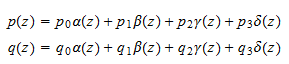Thus, the two coordinate vectors areTheir sum is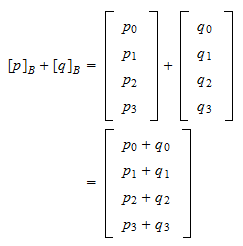so thatThis is the same result that we obtain by carrying out the addition directly:### Exercise 2

Letbe the space of allvectors.

Consider the basiswhereFind the coordinate vector ofwith respect to the given basis.

Solution

We have thatTherefore, the coordinate vector ofis